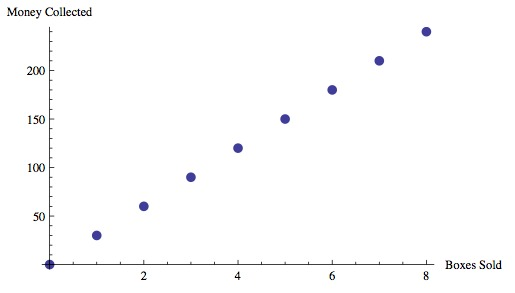# Chocolate Bar Sales

Alignments to Content Standards: 6.EE.C.9

Stephanie is helping her band collect money to fund a field trip. The band decided to sell boxes of chocolate bars. Each bar sells for \$1.50 and each box contains 20 bars. Below is a partial table of monies collected for different numbers of boxes sold. Boxes Sold$b$Money Collected$m$1 \$30.00
2
3
4
5 \$150.00 6 7 8 1. Complete the table above for values of$m$. 2. Write an equation for the amount of money,$m$, that will be collected if$b$boxes of chocolate bars are sold. Which is the independent variable and which is the dependent variable? 3. Graph the equation using the ordered pairs from the table above. 4. Calculate how much money will be collected if 100 boxes of chocolate bars are sold. 5. The band collected \$1530.00 from chocolate bar sales. How many boxes did they sell?

## IM Commentary

In this task students use different representations to analyze the relationship between two quantities and to solve a real world problem. The situation presented provides a good opportunity to make connections between the information provided by tables, graphs and equations. In the later part of the problem, the numbers are big enough so that using the formula is the most efficient way to solve the problem; however, creative use of the table or graph will also work.

This task could be used in a variety of ways: (1) To introduce the idea of independent and dependent variables and to provide a real world example students can use to make connections between different representations. (2) As group work with an extended period of time to compare different solutions. (3) As a formative assessment. Since the task increases in complexity from straight forward to more demanding, it might provide a good opportunity to learn about the studentsâ€™ skills and understanding of the concepts.

## Solutions

Solution: Table, Formula, Graph

1. From the first row in the table, we see that the money collected, $m$, for one box sold, $b$, is \$30.00 since there are 20 candy bars in the box, which cost \$1.50 each. So, we multiply the number of boxes sold by 30 to find the amount of money collected. We fill in the remaining parts of the table as follows:

Boxes Sold ($b$) Money Collected ($m$)
1 \$30.00 2 \$60.00
3 \$90.00 4 \$120.00
5 \$150.00 6 \$180.00
7 \$210.00 8 \$240.00

Note: We can arrive at the same amounts by repeatedly adding \$30.00 as we move down from the first row, effectively adding \$30.00 on for each box sold. As our values for $m$ and $b$ get very large in later parts of this task, addition becomes unrealistic, and multiplication is the easiest method.

2. Using the information we found in part (a) that the amount of money collected, $m$, is equal to \$30 multiplied by the number of boxes sold,$b$, we arrive at the following equation: $$m = 30b$$ Since the equation represents$m$in terms of$b$,$m$is the dependent variable and$b$the independent variable. In this case, we are thinking about the situation as if the amount of money collected depends on the number of boxes that are sold; this interpretation makes sense as the students are first selling the chocolate and then finding how much money they made based on how much they sold. However, we could also write the equation $$b = m/30$$ and think of the number of boxes sold as depending on the amount of money raised. This is especially salient when we get to questions like the one in part (e); it is easy to imagine groups with different fundraising goals asking how many boxes they will have to sell to reach their target. 3. If we plot the ordered pairs$(b, m)$from the completed table in part (a), we obtain the graph below.4. By substituting$b = 100$into our equation from part (b), we see that $$m = 30(100) = 3000$$ and so \$3,000.00 will be collected if 100 boxes of chocolate bars are sold.

5. We will still use our equation from part (b) for this problem, but in this situation $m$ is known, and we will be solving for $b$. Substituting $m = 1530$, we see that

\begin{align} 1530 &= 30b \\ \frac{1530}{30} &= b \\ 51 &= b \end{align}

Thus, the band sold 51 boxes of chocolate bars.

Solution: Table Only

It would be possible to solve the the last two parts of the problem with a table only.

1. See above
2. See above
3. See above
4. We can use the pattern of adding \$30 for each additional box until we get to 10 boxes and then continue in multiples of 10 boxes. Once we have the cost for 20 and 30 boxes we get 50 boxes, and we can double the amount for 50 boxes to get to 100 boxes. Boxes Sold ($b$) Money Collected ($m$) 1 \$30.00
2 \$60.00 3 \$90.00
4 \$120.00 5 \$150.00
6 \$180.00 7 \$210.00
8 \$240.00 9 \$270.00
10 \$300.00 20 \$600.00
30 \$900.00 50 \$1500.00
100 \$3000.00 5. We already have a table entry that shows that 50 boxes cost \$1500 and one more box is an additional \\$30. Therefore, the band sold 51 boxes.